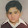Convert String List Data Structure in c and c++ to Struct List Data Structure 11

Shows how to convert string list data structure to a struct list data structure in c and c plus plus programs.

C code:

#include＜stdio.h＞

typedef struct File_element
{
char line;
}file_element;

typedef struct List
{
//char *data;
file_element *data;
struct List *next;
}list;

int main()
{
char *in_file = “test.txt”;
char *out_file = “test.txt.out”;

FILE *input_file = fopen(in_file, “r”);//r for read
FILE *output_file = fopen(out_file, “w”);//w for write

list *elements = NULL;
list *iter =NULL;
list *node = NULL;

char line;
for (; fgets(line, sizeof(line), input_file) != NULL;)
{
iter = malloc(sizeof(list));
//iter-＞data = malloc(strlen(line));//lenght of string which varies
iter-＞data = malloc(sizeof(file_element));//size must be this
//in case struct has more than one members

file_element element ;
*element.line = malloc(sizeof(file_element));//
strcpy(element.line, line);//

//strcpy(iter-＞data, line);//
*iter-＞data =…

c++ programs1.Arif Mahmood says: